http://www.spinellis.gr/pubs/jrnl/1997-AOR-Anneal/html/anneal.html This is an HTML rendering of a working paper draft that led to a publication. The publication should always be cited in preference to this draft using the following reference: Diomidis Spinellis and Chrissoleon T. Papadopoulos. A simulated annealing approach for buffer allocation in reliable production lines. Annals of Operations Research, 93:373–384, 2000. (doi:10.1023/A:1018984125703) Citation(s): 104 (Google Scholar), 58 (selected).This document is also available in PDF format.The document's metadata is available in BibTeX format. This material is presented to ensure timely dissemination of scholarly and technical work. Copyright and all rights therein are retained by authors or by other copyright holders. All persons copying this information are expected to adhere to the terms and constraints invoked by each author's copyright. In most cases, these works may not be reposted without the explicit permission of the copyright holder.

# A Simulated Annealing Approach for Buffer Allocation in Reliable Production Lines

Diomidis Spinellis
Department of Mathematics
GR-832 00 Karlovasi, Samos

GR-821 00 Chios Island

University of the Aegean
Greece
e-mail: {dspin,hpap}@aegean.gr

February, 1998

### Abstract:

We describe a simulated annealing approach for solving the buffer allocation problem in reliable production lines. The problem entails the determination of near optimal buffer allocation plans in large production lines with the objective of maximizing their average throughput. The latter is calculated utilizing a decomposition method. The allocation plan is calculated subject to a given amount of total buffer slots in a computationally efficient way.

# 1 Introduction and Literature Review

Buffer allocation is a major optimization problem faced by manufacturing systems designers. It has to do with devising an allocation plan for distributing a certain amount of buffer space among the intermediate buffers of a production line. This is a very complex task that must account for the random fluctuations in mean production rates of the individual workstations of the lines. To solve this problem there is a need of two different tools. The first is a tool that calculates the performance measure of the line which has to be optimized (e.g., the average throughput or the mean work-in-process). This may be an evaluative method such as simulation, a decomposition method [Ger87,DF93], or a traditional Markovian state model in conjunction with an exact numerical algorithm like [HPB93]. The second tool is a search (generative) method that tries to determine an optimal or near optimal value for the decision variables, which in our case are the buffer capacities of the intermediate buffer locations in the line. Examples of such methods are the classical search methods such as the well-known Hooke-Jeeves method, various heuristic methods, knowledge based methods, and genetic algorithms.

There are some advantages and disadvantages of the various evaluative methods used for modelling production lines. For example, simulation can handle production lines with more realistic processing times but it is a time-consuming method that cannot be utilized in conjunction with a search method that needs to apply it many times. The Markovian state model, on the other hand, provides with an exact solution for small lines (with up to twelve stations), but requires the processing time distribution to be of phase-type. This is not an overly restrictive assumption, because any distribution with a rational Laplace transform can be represented by these distributions with any desired accuracy. The Markovian model has been used to obtain some insights of the problem under investigation. Finally, the decomposition method, works well for large systems (with well over than 50 stations), and it is also applicable to exponentially distributed service times.

As far as the search methods are concerned, the traditional segmentation methods (such as the well-known Hooke-Jeeves method) fail to provide with an optimal solution in all cases, whereas, the various heuristic methods reported in the literature have the advantage of being very fast but are in general inaccurate. Lately, genetic algorithms have been applied to solve the buffer allocation problem. The accuracy and the efficiency of these algorithms have to be tested to proceed with an assessment.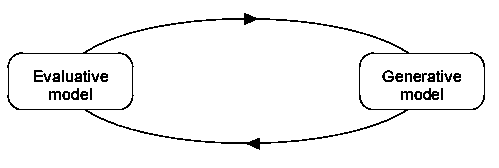Evaluative and generative (optimization) models can be combined in a closed loop' configuration by using feedback from an evaluative model to modify the decision taken by the generative model as illustrated in Figure 1. In this configuration the evaluative model is used to obtain the value of the objective function for a set of inputs. The value of the objective function is then communicated to the generative model which uses it as an objective criterion in its search for an optimal solution. In the rest of this paper we will use the formalism $S(G, E)$ to describe a closed loop system using the generative method $G$ and the evaluative method $E$. The generative models that will be used in this paper are:

CE
complete enumeration,
RE
reduced enumeration, and
SA
simulated annealing.
Furthermore, the evaluative models that will be used:
Exact
the exact numerical algorithm [HPB93], and
Deco
the decomposition algorithm numbered as A3 in [DF93].

For a systematic review of the existing literature in the area of evaluative and generative models of manufacturing systems, the interested reader is addressed, respectively, to two review papers by [DG92] and [PH96] and to the books by [PHB93], [AS93], [BS93], [Ger94], [Per94] and [Alt97], among others.

Although several researchers have studied the problem of optimizing buffer allocation to maximize the efficiency of a reliable production line, there is no method that can handle this problem for large production lines, in a computationally efficient way (see for example, [HS91], and [HSB93]). These methods are based on comprehensive studies to characterize the optimal buffer allocation pattern. Authors have provided extensive numerical results for balanced lines with up to 6 stations and limited results for lines with up to 9 stations.

Other relevant studies are: [CMMT88], who used simulation to investigate the buffer allocation problem, [Pow92], who studied the buffer allocation problem for unbalanced production lines, and [So97], who presented a heuristic method for determining a near optimal buffer allocation in production lines. The differentiation of So's work from the others was that the objective was to minimize the average work-in-process, provided a minimum required throughput is attained.

Furthermore, [BDI95] applied genetic algorithms for the buffer allocation in asynchronous assembly systems.

The objective of this paper is to present a search method for solving the buffer allocation problem in large reliable, balanced and unbalanced, production lines with computational efficiency. The proposed method is a simulated annealing approach that works in close cooperation with a decomposition method as given in [DF93].

Simulated annealing is an adaptation of the simulation of physical thermodynamic annealing principles described by [MRR+53] to the combinatorial optimization problems [KJV83,Cer85]. Similar to genetic algorithms [Hol75,Gol89] and tabu search techniques [Glo90] it follows the local improvement'' paradigm for harnessing the exponential complexity of the solution space.

The algorithm is based on randomization techniques. An overview of algorithms based on such techniques can be found in [GSB94]. A complete presentation of the method and its applications can be found in [LA87] and accessible algorithms for its implementation are presented by [CMMR87,PFTV88]. A critical evaluation of different approaches to annealing schedules and other method optimizations are given by [Ing93].

As a tool for operational research simulated annealing is presented by [Egl90], while [KAJ94] provide a complete survey of simulated annealing applications to operations research problems.

This paper is organized as follows. Section 2 states the problem and the assumptions of the model, whereas, section 3 describes the proposed simulated annealing approach. In section 4, we provide numerical results obtained from the algorithm. Finally, section 5 concludes the paper and suggests some future research directions.

# 2 Assumptions of the Model and the Buffer Allocation Problem

In asynchronous production lines, each part enters the system from the first station, passes in order from all stations and the intermediate buffer locations and exits the line from the last station. The flow of the parts works as follows: in case a station has completed its processing and the next buffer has space available, the processed part is passed on. Then, the station starts processing a new part that is taken from its input buffer. In case the buffer has no parts, the station remains empty until a new part is placed in the buffer. This type of line is subject to manufacturing blocking (or blocking after service) and starving.

Assumptions of the model: It is assumed that the first station is never starved and the last station is never blocked. The processing (service) times at each station are assumed to be independent random variables following the exponential distribution, with mean service rates, $μ$i , $i=1,2,&ldots;, K$. In our model, the stations of the line are assumed to be perfectly reliable, that is, breakdowns are not allowed.

The exponentiality of the processing times as well as the absolute reliability of the line's workstations are rather unrealistic assumptions. However, the service completion times can be exponential or can be approximated by an exponential distribution. The variability in completion times may be attributed to failures and repairs which implicitly exist in the problem at hand. Following this view, the proposed model may be applied to any unreliable production line under the exponentiality assumptions for the service completion times.

Figure 2 depicts a $K$-station line that has $K-1$ intermediate locations for buffers, labelled $B$2, B3, &ldots;, BK .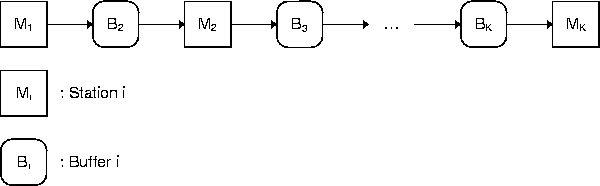The basic performance measures in the analysis of production lines are the average throughput (or mean production rate) and the average work-in-process (WIP) or equivalently the average production (sojourn) time.

The object of the present work is the buffering of asynchronous, reliable production lines with the assumptions given above. The objective is the maximization of the line's throughput, subject to a given total buffer space.

The buffer allocation problem: In mathematical terms, our problem can be stated as follows:

P
Find B=(B2,B3,&ldots;,BK) so as to

  maxOK(B) (1)
subject to: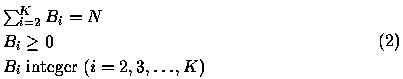where: $N$ is a fixed nonnegative integer, denoting the total buffer space available in the production line.

B=(B2,B3,&ldots;,BK) is the buffer vector', i.e., a vector with elements the buffer capacities of the $K-1$ buffers.

$O$K , denotes the average throughput of the $K$-station line. This is a function of the mean service rates of the $K$ stations, $μ$i,   (i=1,2,&ldots;,K) , of the coefficients of variation, $CV$i , of the service times and the buffer capacities, $B$i .

Methodology of investigation: To solve the optimal buffer allocation problem (P), we have performed the following steps:

S1
We utilized the decomposition method given by [DF93], as an evaluative tool, to determine the mean throughput of the lines. The algorithm computes approximately the throughput for any $K$-station line with finite intermediate buffers and exponentially distributed processing times. The number of feasible allocations of $N$ buffer slots among the $K-1$ intermediate buffer locations increases dramatically with $N$ and $K$ and is given by the formula: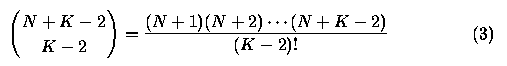S2
To find the buffer allocation that maximizes the throughput of the line, we utilized the simulated annealing method specifically adapted for solving this problem. Our approach is described in detail in the following section.

# 3 The Simulated Annealing Approach

Simulated annealing is an optimization method suitable for combinatorial minimization problems. Such problems exhibit a discrete, factorially large configuration space. In common with all paradigms based on local improvements'' the simulated annealing method starts with a non-optimal initial configuration (which may be chosen at random) and works on improving it by selecting a new configuration using a suitable mechanism (at random in the simulated annealing case) and calculating the corresponding cost differential ( $ΔO$K ). If the cost is reduced, then the new configuration is accepted and the process repeats until a termination criterion is satisfied. Unfortunately, such methods can become trapped'' in a local optimum that is far from the global optimum. Simulated annealing avoids this problem by allowing uphill'' moves based on a model of the annealing process in the physical world.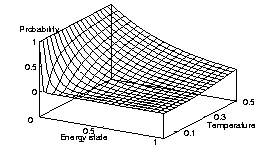Matter can be brought into a low-temperature ground state by careful annealing. First the substance is melted, then it is gradually cooled with a lot of time spent at temperatures near the freezing point. If this procedure is not followed the resulting substance may form a glass with not crystalline order and only metastable, locally optimal structures [KJV83]. During the cooling process the system can escape local minima by moving to a thermal equilibrium of a higher energy potential based on the probabilistic distribution $w$ of entropy $S$

 $S = k lnw$ (4)
where $k$ is Boltzmann's constant and $w$ the probability that the system will exist in the state it is in relative to all possible states it could be in. Thus given entropy's relation to energy $E$ and temperature $T$

 $dS = {d¯ ET}$ (5)
we arrive at the probabilistic expression $w$ of energy distribution for a temperature $T$

 $w ∼exp({-EkT})$ (6)
This so-called Boltzmann probability distribution is illustrated in Figure 3. The probabilistic uphill'' energy movement that is made possible avoids the entrapment in a local minimum and can provide a globally optimal solution.

The application of the annealing optimization method to other processes works by repeatedly changing the problem configuration and gradually lowering the temperature until a minimum is reached.

The correspondence between annealing in the physical world and simulated annealing as used for optimal buffer allocation is outlined in Table 1. The algorithm used for the production line buffer allocation is given in Figure 4.

 Physical World OBA Simulated Annealing Atom placement Line configuration Random atom movements Buffer space movement Energy Throughput Energy differential Configuration throughput differential Energy state probability distribution Changes according to the Metropolis criterion, $exp({-ΔET}) > rand (0 &ldots;1)$, implementing the Boltzmann probability distribution. Temperature Variable for establishing configuration acceptance termination

 1. $[$Set initial line configuration.$]$ Set $B$i ←⌊N/K ⌋ , set $B$K/2 ←BK/2 + N - ∑i=2K ⌊N/K ⌋ . 2. $[$Set initial temperature $T$0 .$]$ Set $T ←0.5$. 3. $[$Initialize step and success count.$]$ Set $S ←0$, set $U ←0$. 4. $[$Create new line with a random redistribution of buffer space.$]$ Move $R$n space from a source buffer $B$Rs to a destination buffer $B$Rd : set B' ←B , set $R$s ←⌊rand [2 &ldots;K+1)⌋ , set $R$d ←⌊rand [2 &ldots;K+1)⌋ , set $R$n ←⌊rand [0 &ldots;BRs+1)⌋ , set $B$R>s ←BRs - Rn , set $B$Rd ←BRd + Rn . 5. $[$Calculate energy differential.$]$ Set $ΔE ← O$K(B) - OK(B') . 6. $[$Decide acceptance of new configuration.$]$ Accept all new configurations that are more efficient and, following the Boltzmann probability distribution, some that are less efficient: if $ΔE < 0$ or $exp({-ΔET}) > rand (0 &ldots;1)$, set B ←B' , set $U ←U + 1$. 7. $[$Repeat for current temperature$]$. Set $S ←S + 1$. If $S < maximum number of steps$, go to step 4. 8. $[$Lower the annealing temperature.$]$ Set $T ←c T$ ($0 < c < 1$). 9. $[$Check if progress has been made.$]$ If $U > 0$, go to step 3; otherwise the algorithm terminates.

# 4 Numerical Results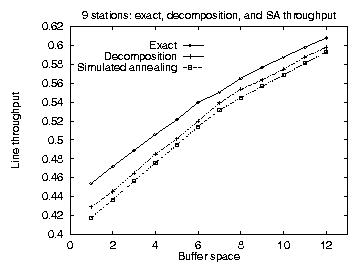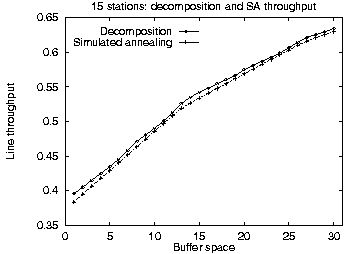In order to evaluate the applicability of the simulated annealing method to the buffer allocation problem we designed and implemented a system to calculate the optimum buffer configuration for a given reliable production line using a simulated annealing algorithm. The system takes as input:

• the number of stations in the production line, $K$,
• the available buffer space, $N$, and
• the station mean service rates, $μ$i , $i=1,2,&ldots;, K$.
Based on the above input, the system calculates the buffer allocations B=(B2,B3,&ldots;,BK) for the maximal line throughput. Furthermore, the system is instrumented to provide as part of the solution the throughput of the suggested configuration, as well as the number of different configurations that were tried. The line throughput is used to evaluate the quality of the suggested configuration when compared with the throughput calculated by other methods. The number of different configurations tried, is used as an objective performance criterion, because the configuration evaluation step is the dominant execution time factor and the basic building block of all optimization methods.

The system is based on the simulated annealing algorithm as described in [PFTV88]. The authors do not clarify that, strictly described, their implementation is a simulated quenching [Ing93] algorithm as it uses an exponential cooling schedule. Our implementation, for efficiency reasons, uses the same exponential cooling schedule namely: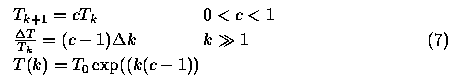instead of the standard logarithmic schedule consistent with the Boltzmann algorithm

 $T(k) = T$0{lnk0lnk} (8)
The random floating point numbers $0 < R < 1$ used for selecting energy differentials based on the annealing temperature $R < exp({-ΔET})$ are produced using the substractive method algorithm described in [Knu81]. Finally, the evaluative function that we used for calculating $ΔE$ is based on the decomposition method [DF93].

In order to evaluate our method's applicability in selecting line configurations we run a number of tests on both balanced and unbalanced lines and compared the simulated annealing results against the results obtained by other methods. For short lines and limited buffer space a complete enumeration of all configurations provided an accurate measure when comparing with the simulated annealing results. For larger configurations we used a reduced enumeration in order to provide the comparative measure.

Reduced enumeration is based on the experimental observation that the absolute difference of the respective elements of the optimal buffer allocation (OBA) vectors with $N$ and $N+1$ buffer slots is less than or equal to 1:

$|B$iN+1 - BiN| ≤1,   ∀i: 2 ≤i ≤K.

In this way, we have been able to derive the OBA by induction for any number $N$ of buffer slots that are to be allocated among the $K-1$ buffer locations of the line. The reduction works as follows: when $N*$ and $K$ are given one needs to determine all the OBA vectors for $N=1,2,&ldots;,N*$ and then for $N=N*+1$ by searching only the values of $B$iN-1 , $B$iN and $B$iN+1 . Furthermore, this reduction starts after a number of total buffer slots $N$. To quantify the reduction, by applying the improved enumeration it has been experimentally observed that the number of iterations were reduced by at least 60% for short lines. This reduction accounts for well over 90% for large production lines (with more than 12 stations). Recall that the number of feasible allocations of $N$ buffer slots among the $K-1$ intermediate buffer locations increases dramatically with $N$ and $K$ and is given by formula (3).

Both methods are subject to the reduced evaluative accuracy of the decomposition method compared to the Markovian model. In Figure 5 we present the optimum throughput configurations for balanced lines found using the simulated annealing method against the throughput found using complete (for 9 stations) and reduced enumeration techniques. It is apparent that the simulated annealing results follow closely the results obtained by the other methods.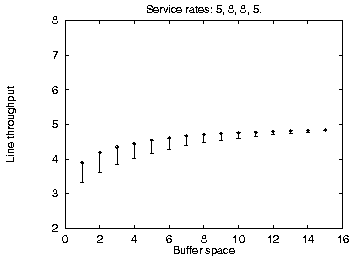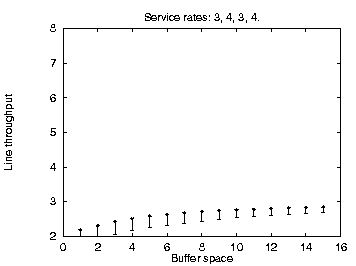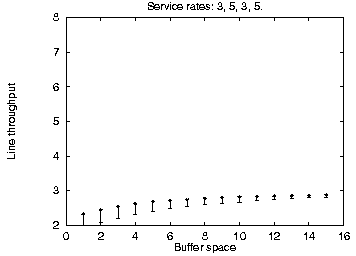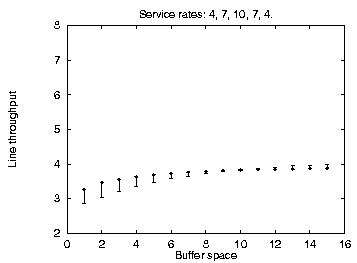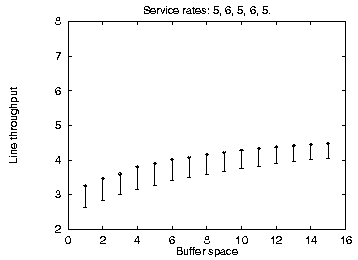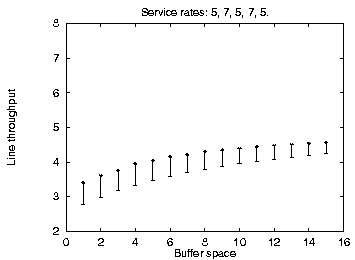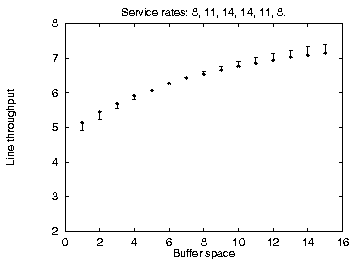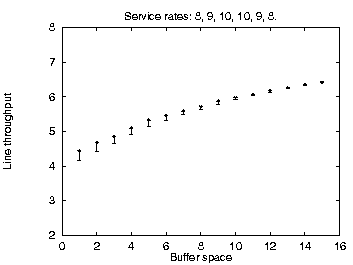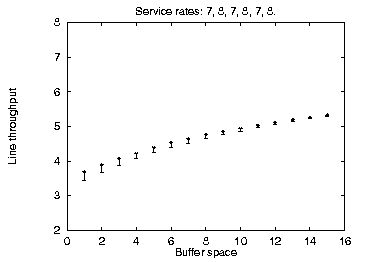In addition to the balanced line evaluation we compared the simulated annealing method against unbalanced line enumeration using the Markovian evaluative procedure for a variety of line sizes, service time configurations, and available buffer space. The results are summarized using error bars in Figure 6. It is apparent that the simulated annealing configurations are not always optimal for limited available buffer space, but they quickly converge on the optimal configurations as buffer space increases. This difference can be accounted by the use of the fast decomposition evaluative procedure used in the similated annealing implementation yielding approximate results against the use of the Markovian evaluative procedure for the enumeration method yielding exact results. As the decomposition method is not accurate for small sets of unbalanced lines this is an expected outcome and could be corrected by using the Markovian evaluation in the simulated annealing optimization of small unbalanced production lines.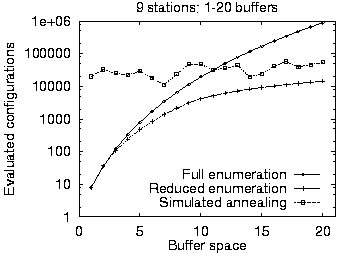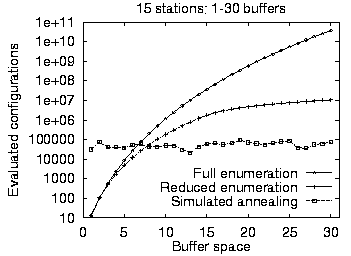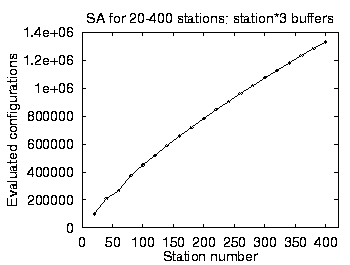Our goal for using the simulated annealing method was to test its applicability to large production line problems where the cost of other methods was prohibitevely expensive. As an example the reduced enumeration method when run on a 15 station line with a buffer capacity of 30 units took more than 10 hours to complete on a 100MHz processor. As shown in Figure 7 the cost of the simulated annealing method is higher than the cost of the full and reduced enumeration methods for small lines and buffer allocations. However, it quickly becomes competitive as the number of stations and the available buffer size increase. Notice that -- in contrast to the other methods -- the simulated annealing cost does not increase together with the available buffer space and that it increases only linearly with the number of stations. The results we obtained could not be verified, because no other numerical results for the buffer allocation problem in large production lines can be found in the open literature.

# 5 Conclusions and Further Work

The results obtained using the simulated annealing method to the reliable line optimal buffer allocation problem are clearly encouraging. The performance and the accuracy of the method, although inferior for optimizing small lines with limited buffer space, seem to indicate clearly that it becomes the method of choice as the problem size increases. These characteristics suggest that the method fits nicely together with the existing optimization tools satisfying the need for a large production line optimization tool.

Further investigation is needed in order to fully evaluate the method's potential. The annealing schedule that we used can clearly be optimized potentially increasing both the method's accuracy and its performance. Methods such as adaptive simulated annealing [Ing89] can be tried on the problem set in order to test their applicability. The use of heuristics in setting up the initial buffer configuration can decrease the number of steps needed for reaching the optimal. Other evaluative methods such as the Markovian model can be used in place of the decomposition algorithm for determining the change differentials. Furthermore, the small nature of changes made to the configuration during the annealing process could be taken into account for optimizing the evaluative procedure. Finally, we would like to test the method's potential on similar problems especially involving parallel station production lines.

# Acknowledgment

We wish to thank Mr. Michael Vidalis for implementing the decomposition algorithm and providing us with the decomposition and reduced decomposition evaluative results.

## References

Alt97
T. Altiok.
Performance Analysis of Manufacturing Systems.
Springer-Verlag, 1997.

AS93
R. G. Askin and C. R. Standridge.
Modeling and Analysis of Manufacturing Systems.
Wiley, 1993.

BDI95
A. A. Bulgak, P. D. Diwan, and B. Inozu.
Buffer size optimization in asynchronous assembly systems using genetic algorithms.
Computers ind. Engng, 28(2):309-322, 1995.

BS93
J. A. Buzacott and J. G. Shanthikumar.
Stochastic Models of Manufacturing Systems.
Prentice Hall, 1993.

Cer85
V. Cerny.
Thermodynamical approach to the traveling salesman problem: an efficient simulation algorithm.
Journal of Optimization Theory and Applications, 45:41-51, 1985.

CMMR87
A. Corana, M. Marchesi, C. Martini, and S. Ridella.
Minimizing multimodal functions of continuous variables with the simulated annealing'' algorithm.
ACM Transactions on Mathematical Software, 13(3):262-280, September 1987.

CMMT88
R. W. Conway, W. L. Maxwell, J. O. McClain, and L. J. Thomas.
The role of work-in-process inventories in series production lines.
Operations Research, 36:229-241, 1988.

DF93
Y. Dallery and Y. Frein.
On decomposition methods for tandem queueing networks with blocking.
Operations Research, 41(2):386-399, 1993.

DG92
Y. Dallery and S. B. Gershwin.
Manufacturing flow line systems: A review of models and analytical results.
Queueing Systems: Theory and Applications, 12:3-94, 1992.

Egl90
R. W. Eglese.
Simulated annealing: A tool for operational research.
European Journal of Operational Research, 46:271-281, 1990.

Ger87
S. B. Gershwin.
An efficient decomposition method for the approximate evaluation of tandem queues with finite storage space and blocking.
Operations Research, 35(2):291-305, 1987.

Ger94
S. B. Gershwin.
Manufacturing Systems Engineering.
Prentice Hall, 1994.

Glo90
F. Glover.
Tabu search -- part I.
ORSA Journal on Computing, 1:190-206, 1990.

Gol89
David E. Goldberg.
Genetic Algorithms: In Search of Optimization & Machine Learning.

GSB94
R. Gupta, S. A. Smolka, and S. Bhaskar.
On randomization in sequential and distributed algorithms.
ACM Computing Surveys, 26(1):7-86, 1994.

Hol75
J. H. Holland.
Adaptation in Natural and Artificial Systems.
University of Michigan Press, Ann Arbor, Michigan, 1975.

HPB93
C. Heavey, H. T. Papadopoulos, and J. Browne.
The throughput rate of multistation unreliable production lines.
European Journal of Operational Research, 68:69-89, 1993.

HS91
F. S. Hillier and K. C. So.
The effect of the coefficient of variation of operation times on the allocation of storage space in production line system.
IIE Transactions, 23:198-206, 1991.

HSB93
F. S. Hillier, K. C. So, and R. W. Boling.
Notes: Toward characterizing the optimal allocation of storage space in production line systems with variable processing times.
Management Science, 39(1):126-133, 1993.

Ing89
L. Ingber.
Very fast simulated re-annealing.
Journal of Mathematical Computation Modelling, 12:967-973, 1989.

Ing93
L. Ingber.
Simulated annealing: Practice versus theory.
Journal of Mathematical Computation Modelling, 18(11):29-57, 1993.

KAJ94
C. Koulamas, S. R. Antony, and R. Jaen.
A survey of simulated annealing applications to operations research problems.
Omega International Journal of Management Science, 22(1):41-56, 1994.

KJV83
S. Kirkpatrick, C. D. Gelatt Jr., and M. P. Vecchi.
Optimization by simulated annealing.
Science, 220:671-679, 1983.

Knu81
D. E. Knuth.
The Art of Computer Programming, volume 2 / Seminumerical Algorithms, pages 171-173.

LA87
P. J. M. Van Laarhoven and E. H. L. Aarts.
Simulated Annealing: Theory and Applications.
D. Reidel, Dordrecht, The Nethelands, 1987.

MRR+53
N. Metropolis, A. N. Rosenbluth, M. N. Rosenbluth, A. H. Teller, and E. Teller.
Equation of state calculation by fast computing machines.
Journal of Chemical Physics, 21(6):1087-1092, 1953.

Per94
H. Perros.
Queueing Networks with Blocking.
Oxford University Press, 1994.

PFTV88
W. H. Press, B. P. Flannery, S. A. Teukolsky, and W. T. Vetterling.
Numerical Recipes in C, pages 343-352.
Cambridge University Press, 1988.

PH96
H. T. Papadopoulos and C. Heavey.
Queueing theory in manufacturing systems analysis and design: A classification of models for production and transfer lines.
European Journal of Operational Research, 92:1-27, 1996.

PHB93
H. T. Papadopoulos, C. Heavey, and J. Browne.
Queueing Theory in Manufacturing Systems Analysis and Design.
Chapman and Hall, 1993.

Pow92
S. G. Powell.
Buffer allocation in unbalanced serial lines.
Working Paper 289, The Amos Tuck School of Business Administration, Dartmouth College, 1992.

So97
K. C. So.
Optimal buffer allocation strategy for minimizing work-in-process inventory in unpaced production lines.
IIE Transactions, 29:81-88, 1997.